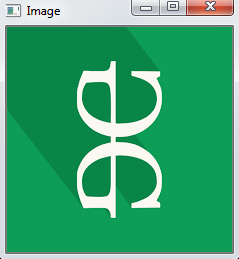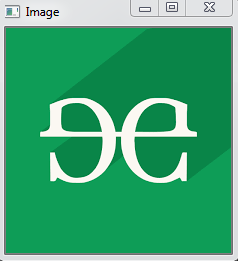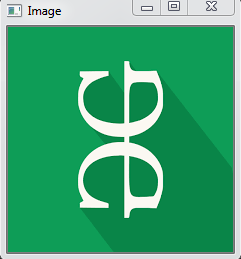# Python OpenCV – cv2.rotate() method

## Python OpenCV – cv2.rotate() method

OpenCV-Python is a library of Python bindings designed to solve computer vision problems. `cv2.rotate()` method is used to rotate a 2D array in multiples of 90 degrees. The function cv::rotate rotates the array in three different ways.

Syntax: cv2.cv.rotate( src, rotateCode[, dst] )

Parameters:
src: It is the image whose color space is to be changed.
rotateCode: It is an enum to specify how to rotate the array.
dst: It is the output image of the same size and depth as src image. It is an optional parameter.

Return Value: It returns an image.

Image used for all the below examples:Example #1: Rotate by 90 degrees clockwise

 `# Python program to explain cv2.rotate() method` ` ` `# importing cv2` `import` `cv2` ` ` `# path` `path ``=` `r``'C:\Users\user\Desktop\geeks14.png'` ` ` `# Reading an image in default mode` `src ``=` `cv2.imread(path)` ` ` `# Window name in which image is displayed` `window_name ``=` `'Image'` ` ` `# Using cv2.rotate() method` `# Using cv2.ROTATE_90_CLOCKWISE rotate` `# by 90 degrees clockwise` `image ``=` `cv2.rotate(src, cv2.cv2.ROTATE_90_CLOCKWISE)` ` ` `# Displaying the image` `cv2.imshow(window_name, image)` `cv2.waitKey(``0``)`

Output:Example #2: Rotate by 180 degrees clockwise

 `# Python program to explain cv2.rotate() method` ` ` `# importing cv2` `import` `cv2` ` ` `# path` `path ``=` `r``'C:\Users\user\Desktop\geeks14.png'` ` ` `# Reading an image in default mode` `src ``=` `cv2.imread(path)` ` ` `# Window name in which image is displayed` `window_name ``=` `'Image'` ` ` `# Using cv2.rotate() method` `# Using cv2.ROTATE_180 rotate by ` `# 180 degrees clockwise` `image ``=` `cv2.rotate(src, cv2.ROTATE_180)` ` ` `# Displaying the image` `cv2.imshow(window_name, image)` `cv2.waitKey(``0``)`

Output:Example #3: Rotate by 270 degrees clockwise

 `# Python program to explain cv2.rotate() method` ` ` `# importing cv2` `import` `cv2` ` ` `# path` `path ``=` `r``'C:\Users\user\Desktop\geeks14.png'` ` ` `# Reading an image in default mode` `src ``=` `cv2.imread(path)` ` ` `# Window name in which image is displayed` `window_name ``=` `'Image'` ` ` `# Using cv2.rotate() method` `# Using cv2.ROTATE_90_COUNTERCLOCKWISE ` `# rotate by 270 degrees clockwise` `image ``=` `cv2.rotate(src, cv2.ROTATE_90_COUNTERCLOCKWISE)` ` ` `# Displaying the image` `cv2.imshow(window_name, image)` `cv2.waitKey(``0``)`

Output:Last Updated on November 13, 2021 by admin

## Reset Index in Pandas DataframeReset Index in Pandas Dataframe

Reset Index in Pandas Dataframe   Let’s discuss how to reset index in Pandas DataFrame.

## Find length of a string in python (4 ways)Find length of a string in python (4 ways)

Find length of a string in python (4 ways) Strings in Python are immutable sequences of Unicode code

## Python – math.sin() functionPython – math.sin() function

Python | math.sin() function In Python, math module contains a number of mathematical operations, which

## Python – os.system() methodPython – os.system() method

Python | os.system() method The OS module in python provides functions for interacting with the

## reduce() in Pythonreduce() in Python

Python reduce() The reduce(fun,seq) function is used to apply a particular function passed in its

## Python Program to Check if Two Strings are AnagramPython Program to Check if Two Strings are Anagram

Python Program to Check if Two Strings are Anagram Question: Given two strings s1 and

## Python – Implementation of Polynomial RegressionPython – Implementation of Polynomial Regression

Python | Implementation of Polynomial Regression Polynomial Regression is a form of linear regression in which

## Timer Objects in PythonTimer Objects in Python

Timer Objects in Python Timer objects are used to represent actions that needs to be